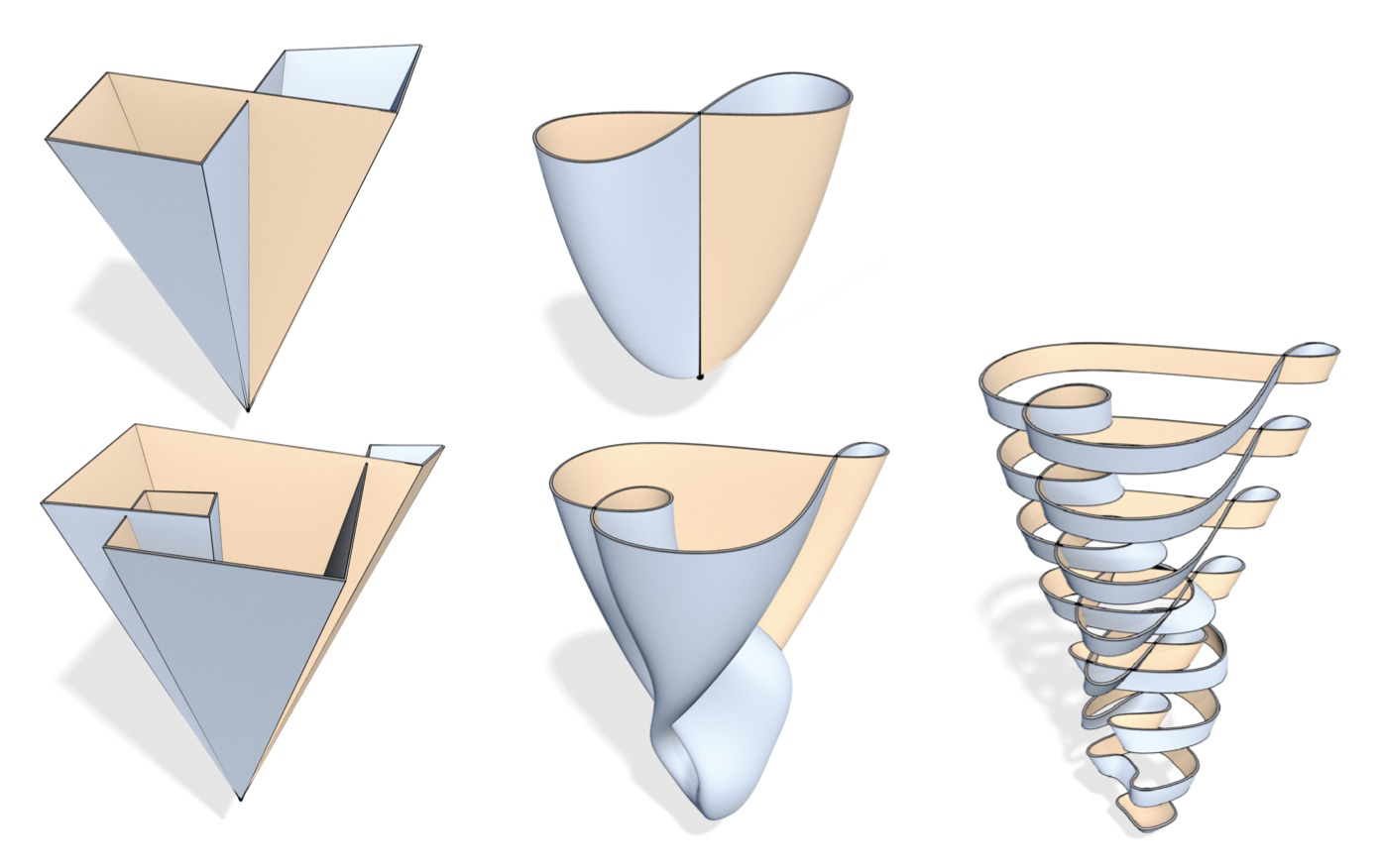## Fall 2020 Geometry/Topology Seminar

Organizers: Joseph Wells, Nur Saglam
Meeting Tuesdays at 3:30pm on Zoom (meeting ID: 943 4713 2523) or in McBryde 563.

 Date Speaker Title & Abstract (click the title to expand) 09/01/2020 David Polletta (ASU) A gentle introduction to complex hyperbolic geometry In this talk, I will discuss some of the building blocks of complex hyperbolic geometry. In doing so, I will point out some differences between real hyperbolic space and complex hyperbolic space including: the various analogous models in both settings, the difference in setup using symmetric bilinear forms and Hermitian forms, and the nature of distance, isometries, and geodesic subspaces. I will also discuss the connection of the boundary at infinity of the complex hyperbolic plane to the Heisenberg group. This introduction is aimed to provide the necessary background materials to discuss how to derive presentations for a special class of lattices in $\mathbf{H}_{\mathbb{C}}^2$ known as the Picard modular groups. 09/08/2020 David Polletta (ASU) Picard modular group presentations In this talk, I will discuss a method for obtaining group presentations for a particular class of lattices in the complex hyperbolic plane known as the Picard modular groups. The method deals with finding an appropriate covering of $\mathbf{H}_{\mathbb{C}}^2$ by translates of a collection of horoballs under the action of a discrete group, $\Gamma$, and appealing to a theorem of Macbeath. We will focus on the application of this method to the case where our lattice has a single cusp, and as examples, we will derive presentations for the Picard modular groups when d = 2 and d =11. The presentations for the Picard modular groups in the cases d=2,11 completes the list of presentations for Picard modular groups with entries from Euclidean domains. We will also discuss how the method changes when our lattice has more than one cusp. 09/22/2020 Nur Saglam (VT) Introduction to Symplectic Manifolds and Mapping Class Groups First, we will give a brief introduction to symplectic manifolds and Lefschetz fibrations. Then we will talk about Mapping Class Groups and their connection with the Lefschetz Fibration. Finally, we will provide examples. This talk will be a preparation for the next talk, which I will talk about my recent work. 09/29/2020 Nur Saglam (VT) Constructions of Lefschetz fibrations using cyclic group actions We construct families of Lefschetz fibrations over $S^2$ using finite order cyclic group actions on the product manifolds $\Sigma_g \times \Sigma_g$ for $g>0$. We also obtain more families of Lefschetz fibrations by applying the rational blow-down operation to these Lefschetz fibrations. This is a joint work with Anar Akhmedov and Mohan Bhupal. 10/20/2020 Josh Clemons (VT) Curvature without a shape operator In this talk I will go through a combinatorial approach to understanding/measuring curvature. It is accessible to undergraduates and particularly interesting for those that want to understand a discrete version of the Gauss-Bonnet Theorem. There are a few related topics that I would like to cover in this short talk. As a theme, my focus will be how I engage undergraduates with topics that sit in the intersection of geometry, topology, and combinatorics. 11/03/2020 Joe Wells (VT) Triangle Groups I - An Introduction and Takeuchi's Classification At one time or another, most have probably found themselves bored in class with a pencil and ruler, doodling tilings of a plane with triangles. These triangular tilings can be realized in terms of group actions, and for a particularly nice family of triangles, we have the so-called "triangle groups." In this talk, I'll motivate triangle groups and we'll explore some of the interplay between the group theory and geometry (possibly with a sprinkling of arithmeticity, if time allows). 11/17/2020 Joe Wells (VT) Triangle Groups II - Beyond Triangles (Arithmetic Hyperbolic Reflection Groups) Last time we looked at groups generated by reflections in the sides of triangles and ultimately were able to reconcile the geometric intuition with the algebra and classify these triangles by arithmeticity. It's then natural to ask if we can play this same game with other (convex) polygons or other higher-dimensional polytopes. As one might expect, keeping track of the geometric features becomes considerably harder as the combinatorial complexity of the polygon increases. Instead, the slightly more natural approach (which generalizes to higher dimensions) is to study which polygons (or polytopes) can arise from a given algebraic construction. In 1975, E. Vinberg produced an algorithm for finding the convex polytopes, and in her 2015 PhD thesis, A. Mark gives an algorithm that improves upon Vinberg's original ideas in the setting of totally real quadratic number fields and obtains some effective bounds. In this talk, I'd like to motivate the algebraic setting and outline Mark's algorithm. Provided time allows, I'd like to also discuss some recent joint work with Mark for extending her algorithm to the more general case of totally real (Galois) number fields.